International
Tables for
Crystallography
Volume B
Reciprocal space
Edited by U. Shmueli

International Tables for Crystallography (2010). Vol. B, ch. 5.2, pp. 648-649   | 1 | 2 |

## Section 5.2.6. Semi-reciprocal space

A. F. Moodie,a J. M. Cowleyb and P. Goodmanc

aDepartment of Applied Physics, Royal Melbourne Institute of Technology, 124 La Trobe Street, Melbourne, Victoria 3000, Australia,bArizona State University, Box 871504, Department of Physics and Astronomy, Tempe, AZ 85287–1504, USA, and cSchool of Physics, University of Melbourne, Parkville, Australia

### 5.2.6. Semi-reciprocal space

| top | pdf |

In the derivation of electron-diffraction equations, it is more usual to work in semi-reciprocal space (Tournarie, 1962). This can be achieved by transforming equation (5.2.2.1)with respect to x and y but not with respect to z, to obtain Tournarie's equation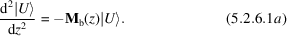Hereis the column vector of scattering amplitudes and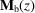is a matrix, appropriate to LEED, with k vectors as diagonal elements and Fourier coefficients of the potential as nondiagonal elements.

This equation is factorized in a manner parallel to that used on the real-space equation [equation (5.2.3.1)] (Lynch & Moodie, 1972) to obtain Tournarie's forward-scattering equationwhereandwhereare the scattering coefficients andare the structure amplitudes in volts. In order to simplify the electron-diffraction expression, the third crystallographic index `l' is taken to represent the periodicity along the z direction.

The double solution involving M of equation (5.2.6.1b) is of interest in displaying the symmetry of reciprocity, and may be compared with the double solution obtained for the real-space equation [equation (5.2.3.2)]. Normally thesolution will be followed through to give the fast-electron forward-scattering equations appropriate in HEED., however, represents the equivalent set of equations corresponding to the z reversed reciprocity configuration. Reciprocity solutions will yield diffraction symmetries in the forward direction when coupled with crystal-inverting symmetries (Section 2.5.3).

Once again we set out to solve the forward-scattering equation (5.2.6.1a,b) now in semi-reciprocal space, and define an operator[compare with equation (5.2.4.1a)] such that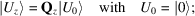i.e.,is an operator that, when acting on the incident wavevector, generates the wavefunction in semi-reciprocal space.

Again, the differential equation can be transformed into an integral equation, and once again this can be iterated. In the projection approximation, withindependent of z, the solution can be written as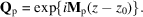A typical off-diagonal element is given by, whereis the angle through which the beam is scattered. It is usual in the literature to find thathas been approximated as unity, since even the most accurate measurements are, so far, in error by much more than this amount.

This expression for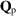is Sturkey's (1957) solution, a most useful relation, written explicitly as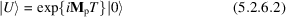with T the thickness of the crystal, and, the incident state, a column vector with the first entry unity and the rest zero.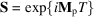is a unitary matrix, so that in this formulation scattering is described as rotation in Hilbert space.

### References

Lynch, D. F. & Moodie, A. F. (1972). Numerical evaluation of low energy electron diffraction intensities. Surf. Sci. 32, 422–438.
Sturkey, L. (1957). The use of electron-diffraction intensities in structure determination. Acta Cryst. 10, 858–859.
Tournarie, M. (1962). Recent developments of the matrical and semi-reciprocal formulation on the field of dynamical theory. J. Phys. Soc. Jpn, 17, Suppl. B11, 98–100.